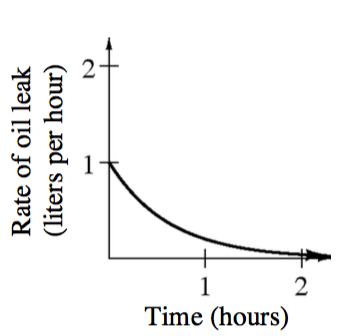### Home > CALC > Chapter 1 > Lesson 1.4.3 > Problem1-167

1-167.

Oil is leaking out of a car at a rate of $y = 0.2^t$ liters/hour for $0 ≤ x ≤ t$.

1. Sketch a graph of this situation. Label the units on the axes.2. Shade the area under the curve for $0 ≤ t ≤ 1$. What does the shaded area represent? What are the units?

Liters per hours multiplied by hours equals __________________ ?

The number of liters that leaked out between $0$ and $1$ hours. The units are liters.